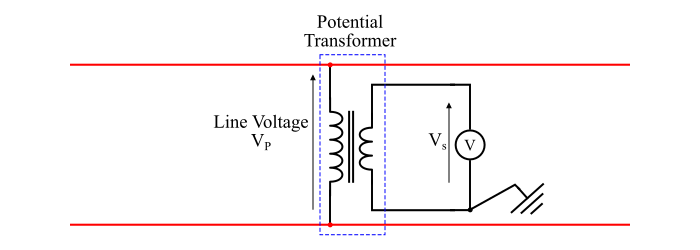# What is Potential Transformer (P.T.) and how it works?

A potential transformer (P.T.) is an instrument transformer which is used for the protection and measurement purposes in the power systems. A potential transformer is mainly used to measure high alternating voltage in a power system.

Potential transformers are step-down transformers, i.e., they have many turns in the primary winding while the secondary has few turns. The figure shows a typical potential transformer for the measurement of high alternating voltage. From the figure, it is clear that a P.T. is a well designed step down transformer.The stepped down voltage by the Potential transformer can be measure using a low range AC voltmeter. The potential transformer has shell type construction of its magnetic core for better accuracy. One end of the secondary winding of the potential transformer is grounded to provide the proper protection to the operator.

The primary winding of the potential transformer is connected across the high voltage power line whose voltage is to be measured and a low-range AC voltmeter (usually 0-110 V) is connected across the secondary winding of the P.T.

The relationship between the line voltage (VP) and the AC voltmeter voltage (VS) is given by,

$$\mathrm{\frac{𝑉_{𝑃}}{𝑉_{𝑆}}=\frac{𝑁_{𝑃}}{𝑁_{𝑆}}}$$

Here, the ratio of primary voltage to the secondary voltage of a potential transformer is known as the potential transformation ratio or P.T. ratio, i.e.

$$\mathrm{P. T.\:ratio =\frac{𝑉_{𝑃}}{𝑉_{𝑆}}}$$

Therefore, the voltage of the power line (VP) is given by,

$$\mathrm{𝑉_{𝑃} = P. T.\:ratio × 𝑉_{𝑆}}$$

Hence, the voltage of the power line is given by the product of the AC voltmeter reading and the P.T. ratio of the potential transformer.

## Numerical Example

A potential transformer of 11000/110 V is used to measure voltage in a transmission line. If the voltmeter indicates 85 V, then what is the voltage in the line?

### Solution

$$\mathrm{Line\:voltage = P. T.\:ratio × Voltmeter\:reading}$$

$$\mathrm{Line\:voltage,\:𝑉 =\frac{11000}{110}× 85 = 8500\:V}$$